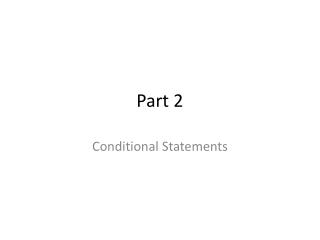DownloadDownload PresentationPart 2

# Part 2

Télécharger la présentation## Part 2

- - - - - - - - - - - - - - - - - - - - - - - - - - - E N D - - - - - - - - - - - - - - - - - - - - - - - - - - -
##### Presentation Transcript

1. Part 2 Conditional Statements

2. Conditional Statements • A conditional is an If – then statement • p  q (read as if p then q or p implies q) • The Hypothesis is the part p following if • The Conclusion is the part q following then.

3. Identifying the Hypothesis and Conclusion • What is the hypothesis and conclusion of the conditional? • If an animal is a robin, then the animal is a bird • H – An animal is a robin • C – The animal is a bird • If an angle measures 130, then the angle is obtuse • H – An angle measures 130 • C – The angle is obtuse

4. Writing a Conditional • Write the following statement as a conditional • Vertical angles share a vertex • Step 1: Identify the Hypothesis and Conclusion • H: Vertical Angles • C: Share a vertex • Step 2: Write the Conditional • If two angles are vertical, then they share a common vertex • You Try: How can you write “Dolphins are mammals” as a conditional? • If an animal is a dolphin, then it is a mammal

5. Truth Value • The truth value of a conditional is either true or false. • To show a conditional is true, show that every time the hypothesis is true, the conclusion is also true • To show a conditional is false find one counter example for which the hypothesis is true and the conclusion is false

6. Finding the Truth Value of a Conditional • Is this conditional true or false, if it is false find a counter example. • If a women is Hungarian, then she is European. • This is True! • If a number is divisible by 3, then it is odd. • This is false, the number 12 is divisible by three and not odd. • If a month has 28 days than it is February • This false, January has 28 days • If two angles form a linear pair, then they are supplementary • True!

7. Negation • The negation of a statement p is the opposite of that statement, the symbol is ~p and is read “not p” • Example: • The negations of the statement “the sky is blue” is “the sky is not blue” • You use the negation to write statements related to a condition

8. Related Conditional Statements

9. Truth Value Equivalent Statements have the same truth value, the conditional and contrapositive are equivalent, and are the converse and inverse statements.

10. You Try • Write the Converse, Inverse and Contrapositive statements • IF a vegetable is a carrot, then it contains beta carotene • Converse • If a vegetable contains beta carotene then it is a carrot • False (Spinach has Beta Carotene) • Inverse • If a vegetable is not a carrot then it does not contain beta carotene • False • Contrapositive • If a vegetable does not contain beta carotene then it is not a carrot • True!